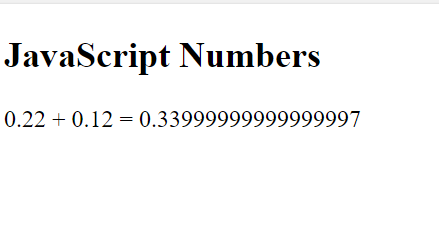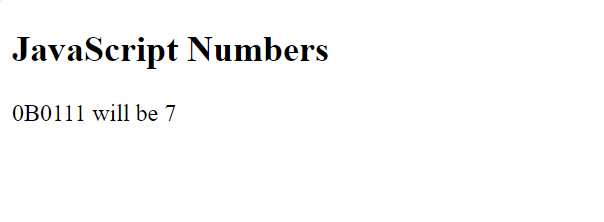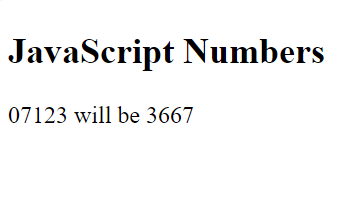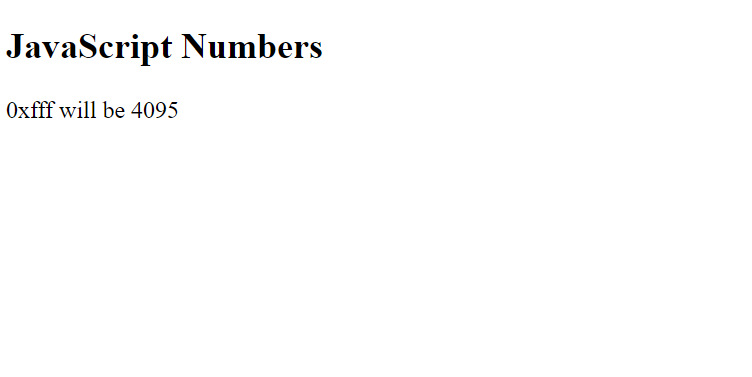# JavaScript | Numbers

• Last Updated : 21 Dec, 2020

JavaScript numbers are always stored as double-precision 64-bit binary format IEEE 754.
This format stores numbers in 64 bits,

• 0-51 bit stores value(fraction)
• 52-62 bit stores exponent
• 63-bit stores sign

The types of number literals You can use decimal, binary, octal, and hexadecimal.

• Decimal Numbers:
JavaScript Numbers does not have different types of numbers(ex: int, float, long, short) which other programming languages do. It has only one type of number and it can hold both with or without decimal values.
```Var a=33;
var b=3.3;
var x = 0562   //x will be 370(parsed as an octal number).```
• If the number starts with 0 and the following number is smaller than 8. It will be parsed as an Octal Number.
Integers are accurate up to 15 digits:
```var a = 999999999999999;   // a will be 999999999999999
var b = 9999999999999999;     // b will be 10000000000000000```
• The floating point is not 100% accurate. The maximum number of decimals is up to 17.
`var x = 0.22 + 0.12;   //x will be 0.33999999999999997`
• Example-1:

## html

 `` `<``html``>`   `<``body``>`   `    ``<``h2``>` `      ``JavaScript Numbers` `  ```   `    ``<``p` `id``=``"num"``>`     `    ``<``script``>` `        ``var x = 0.22 + 0.12;` `        ``document.getElementById(` `          ``"num").innerHTML = ` `          ``"0.22 + 0.12 = " + x;` `    ```   ``   ``

• Output:• Binary Numbers

```var x = 0b11;        // x will be 3
var x = 0B0111;       // x will be 7```
• Example-2:

## html

 `` `<``html``>`   `<``body``>`   `    ``<``h2``>` `      ``JavaScript Numbers` `  ```   `    ``<``p` `id``=``"num"``>`     `    ``<``script``>` `        ``var x = 0 B0111;` `        ``document.getElementById(` `          ``"num").innerHTML = ` `          ``"0B0111 will be " + x;` `    ```   ``   ``

• Output:• Octal Numbers
They start with 0 followed by a number of ranges from 0-7. If any number is used it will be taken as a decimal number.
```var x = 0111;      //x will be 73
var x = 07123;     //x will be 3667```
• Example-3:

## html

 `` `<``html``>`   `<``body``>`   `    ``<``h2``>` `      ``JavaScript Numbers` `  ```   `    ``<``p` `id``=``"num"``>`     `    ``<``script``>` `        ``var x = 07123;` `        ``document.getElementById(` `          ``"num").innerHTML = ` `          ``"07123 will be " + x;` `    ```   ``   ``

• Output:They start with 0x or 0X followed by any digit belonging (0123456789ABCDEF)
`var x = 0xfff;         //x will be 4095`
• Example-2:

## html

 `` `<``html``>`   `<``body``>`   `    ``<``h2``>JavaScript Numbers`   `    ``<``p` `id``=``"num"``>`     `    ``<``script``>` `        ``var x = 0xfff;` `        ``document.getElementById(` `          ``"num").innerHTML = ` `          ``"0xfff will be " + x;` `    ```   ``   ``

• Output:• Exponentiation:
```var x = 2E5   // x will be 200000
var x = 34e3   // x will be 34000
var x = 23e-5 //x will be 0.00023```
• JavaScript uses the + operator for both addition and concatenation.
```var a = 20;
var b = 20;
var c = a + b;           // c will be 40 (a number)```
• Concatenation:
```var a = "10";
var b = "20";
var c = a + b;           // c will be 1020 (a string)```
• Numeric Strings
NaN is a reserved word indicating not a number.
``` var x= "12345" ;        // x will be a string(NaN)
var x = 67 ;            // x will be number```

My Personal Notes arrow_drop_up
Recommended Articles
Page :